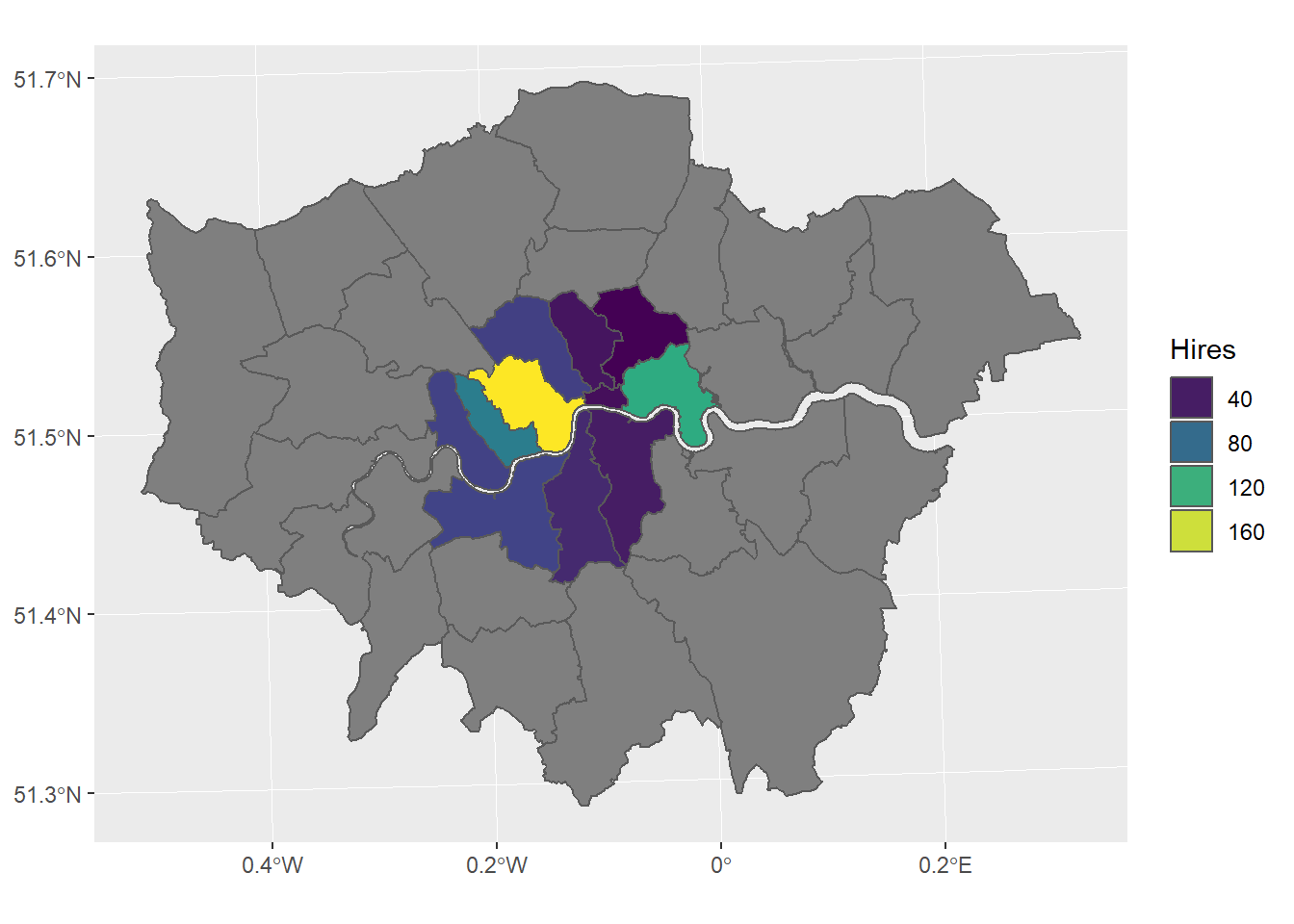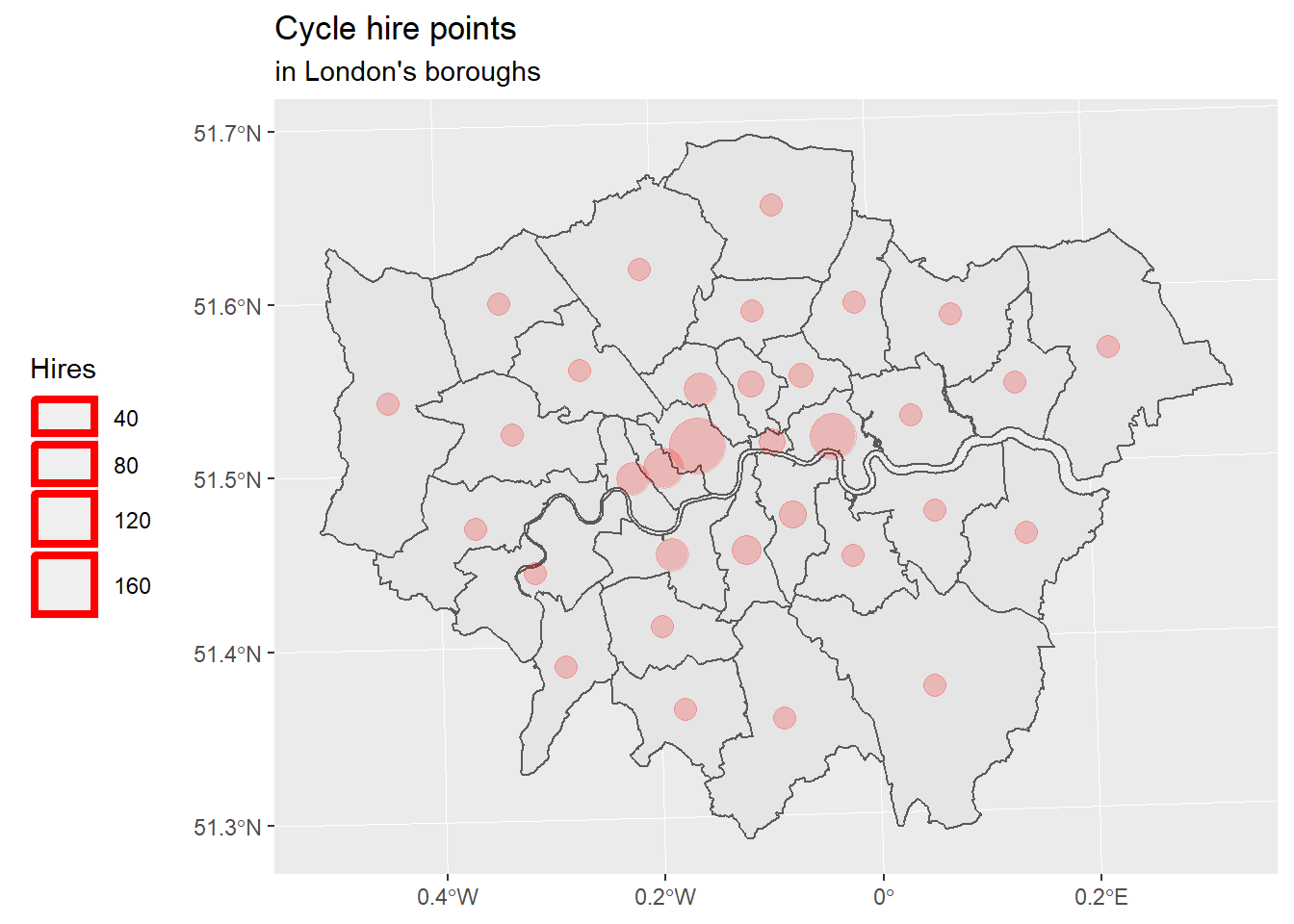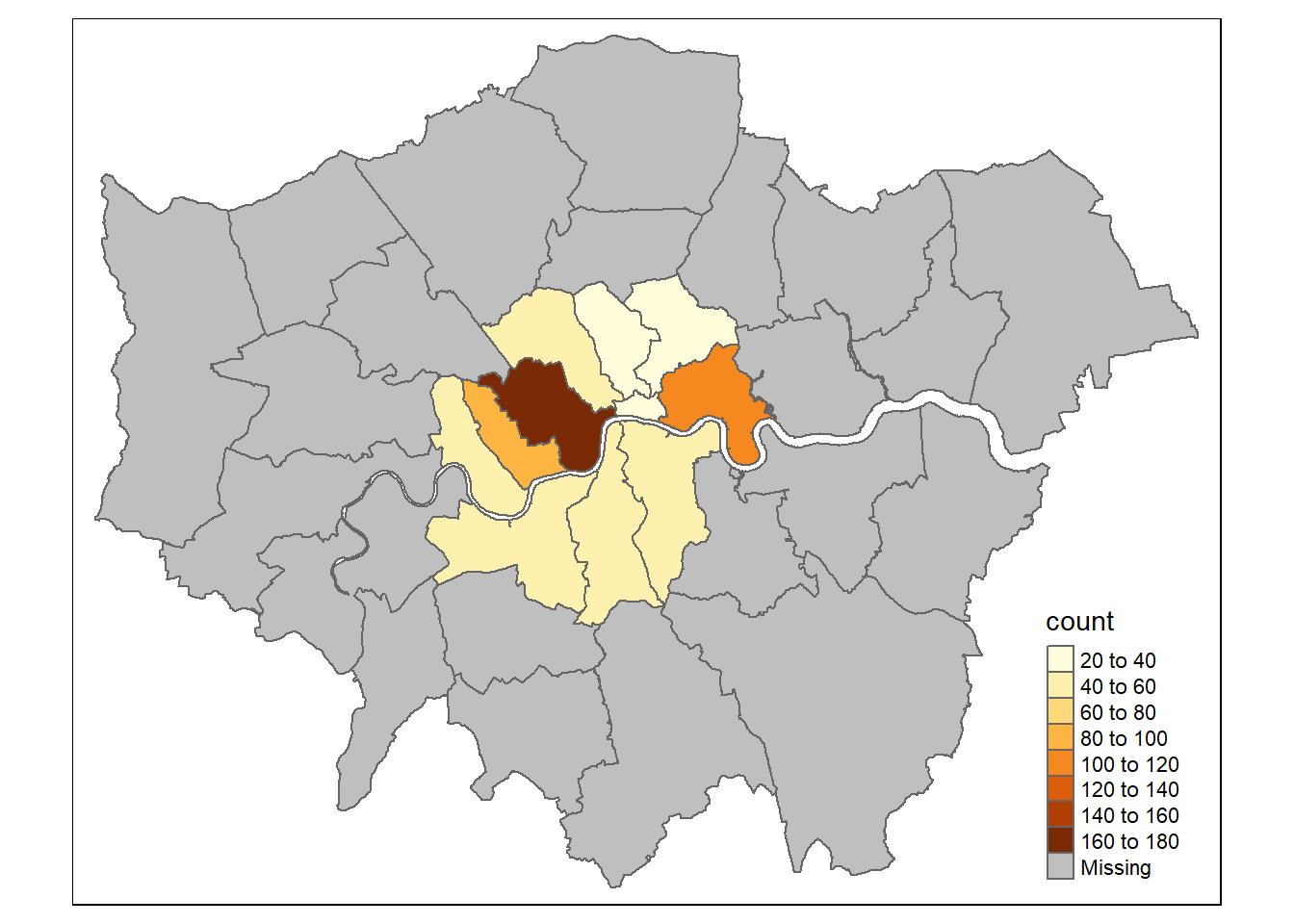# Chapter 5 Maps are plots !

We need to visualise our data. Visualizing geospatial data often means maps and maps are plots ! (In R) You can use plotting tools like `plot` or `ggplot2`, they all accept spatial dataframes. There is also packages to help mapping like {tmap} and {cartography}.

So let’s see how we do plots in R ! First we need data.

## 5.1 Prepare the work

### 5.1.1 Libraries

``````library(sf)
library(here)
library(dplyr)
library(ggplot2)
library(ggspatial)
library(tmap)``````

### 5.1.2 Data

Let’s see what data we stored previously.

``st_layers(here("foss4g_R_workshop.gpkg"))``
``````Driver: GPKG
Available layers:
layer_name geometry_type features fields
1    london_boroughs_27700 Multi Polygon       33      9
2 boroughs_centroids_27700         Point       33      4
3         cycle_hire_27700         Point      742      9``````

We can see our 3 datasets. We can loaded them into memory.

``````cycle_hire_27700 <- st_read(
dsn = here("foss4g_R_workshop.gpkg"),
layer = "cycle_hire_27700")``````
``````Reading layer `cycle_hire_27700' from data source `D:\Roelandt\PERSONNEL\FOSS4G2019_Geoprocessing_with_R_workshop\foss4g_R_workshop.gpkg' using driver `GPKG'
Simple feature collection with 742 features and 9 fields
geometry type:  POINT
dimension:      XY
bbox:           xmin: 522502 ymin: 174408 xmax: 538733.2 ymax: 184421
epsg (SRID):    NA
proj4string:    +proj=tmerc +lat_0=49 +lon_0=-2 +k=0.9996012717 +x_0=400000 +y_0=-100000 +ellps=airy +towgs84=446.448,-125.157,542.06,0.15,0.247,0.842,-20.489 +units=m +no_defs``````
``````london_boroughs_27700 <- st_read(
dsn = here("foss4g_R_workshop.gpkg"),
layer = "london_boroughs_27700")``````
``````Reading layer `london_boroughs_27700' from data source `D:\Roelandt\PERSONNEL\FOSS4G2019_Geoprocessing_with_R_workshop\foss4g_R_workshop.gpkg' using driver `GPKG'
Simple feature collection with 33 features and 9 fields
geometry type:  MULTIPOLYGON
dimension:      XY
bbox:           xmin: 503568.2 ymin: 155850.8 xmax: 561957.5 ymax: 200933.9
epsg (SRID):    NA
proj4string:    +proj=tmerc +lat_0=49 +lon_0=-2 +k=0.9996012717 +x_0=400000 +y_0=-100000 +ellps=airy +towgs84=446.448,-125.157,542.06,0.15,0.247,0.842,-20.489 +units=m +no_defs``````
``````boroughs_centroids_27700<- st_read(
dsn = here("foss4g_R_workshop.gpkg"),
layer = "boroughs_centroids_27700")``````
``````Reading layer `boroughs_centroids_27700' from data source `D:\Roelandt\PERSONNEL\FOSS4G2019_Geoprocessing_with_R_workshop\foss4g_R_workshop.gpkg' using driver `GPKG'
Simple feature collection with 33 features and 4 fields
geometry type:  POINT
dimension:      XY
bbox:           xmin: 507889.7 ymin: 163541.2 xmax: 554049 ymax: 196420.8
epsg (SRID):    NA
proj4string:    +proj=tmerc +lat_0=49 +lon_0=-2 +k=0.9996012717 +x_0=400000 +y_0=-100000 +ellps=airy +towgs84=446.448,-125.157,542.06,0.15,0.247,0.842,-20.489 +units=m +no_defs``````

## 5.2 The `plot()` way

You can plot any spatial dataframe. It simply works but the syntax is no easy. We did one example in 4.2. There is several examples online if you want to go further.

## 5.3 Using {ggplot}

Since we are using a lot of {tidyverse} compatible package, it is logical to use {ggplot2} which is part of this toolset. The basic philosophy of ggplot is layers, first you create an empty plot with the data then you add a geometry (`geom_*()`) and some aesthetics (`aes()`) for that geometry. You can add multiple layers and add more informations (labels, titles). All those elements are linked by a `+` symbol. It is built on A Layered Grammar of Graphics (Wickham 2010).

``````london_boroughs_27700 %>% # pipe data to
ggplot() +                # a ggplot function
geom_sf(                # precise that it will be a spatial geometry
aes(                  # provide some aesthetics
geometry = geom,    # the geometry column (usually auto detected)
fill = count)       # we want the polygon color to change following the count
) -> g # store it in g

g # display g``````We can customize our map by adding thing to it. For example a viridis color scale (friendly to common forms of colorblindness).

``````g <- g +
scale_fill_viridis_c(
guide = guide_legend(title = "Hires") # legend title
)
g``````We can set a theme and add titles

``````g <- g +
theme_bw() +
ggtitle("Cycle hire points", subtitle = "in London's boroughs")
g````````````ggplot() +  geom_sf(data = london_boroughs_27700) + # add boroughs shape to the map
geom_sf(data = boroughs_centroids_27700, # add the boroughs centroids>
aes(size = boroughs_centroids_27700\$count), # fix size of points (by area)
color = 'red', alpha = 1/5)+ # set points colour and transparency
ggtitle("Cycle hire points", subtitle = "in London's boroughs") + # set the map title
theme(legend.position = 'left') + # Legend position
scale_size_area(name = 'Hires',max_size=10) # 0 value means 0 area + legend title``````Well, the legend is not convincing me.Although it should work like in the OSGeoLive R Quickstart.

And if we want to had decorations (scale bar, north arrow), we need to use a another package: {ggspatial}.

Now, let’s use proper packages for cartography. First {tmap} who was created before {ggplot} was able to do maps with {sf} objects. Then {cartography} who is a based on `plot()` from base R.

## 5.4 Using {tmap}

{tmap} was created to help people mapping when {ggplot2} was not able to plot {sf} objects3

``````library(tmap)

tm_shape(london_boroughs_27700) +
tm_polygons("count")``````We can see that there is a color scale for the quantity of hire points.

One cool thing with {tmap}, it is that you can use 2 modes: “plot” and “view”. The “view” mode is an interactive one ! Try to go over or click on polygons !

``````tmap_mode("view")

tm_shape(london_boroughs_27700) +
tm_polygons("count")``````